# Analyze of PyPy warmup in performance benchmarks¶

## Goal¶

The purpose of this analysis if to choose the number of warmups and samples for performance benchmarks for PyPy 5.7 on the speed-python server.

• small enough to reduce total benchmark runtime

• get a mean-stdev of the sample close enough to mean-stdev of all values

• if performance has a cycle (usually with a spike at the start or end): compute 5 cycles to use the average of a cycle

## General notes¶

The analysis is based on a single data set: pypy2_571_warmups.json.gz.

• PyPy2 5.7.1 (revision 1aa2d8e03cdf): 64-bit, static binary

• perf 1.2 (dev), performance 0.5.5 (dev)

• performance hacked to run exactly 10 worker processes, each computes 250 values with 0 warmup

• Date: 2017-04-13 22:19 - 2017-04-14 09:01 (10h45)

• 67 benchmarks

After computing these data, the following 4 microbenchmarks have been removed from performance benchmark suite and so will be ignored here.

• call_method

• call_method_slots

• call_method_unknown

• call_simple

speed-python server:

• cpu: 2 HP DL380 G7 Intel® Xeon® X5680 (3.33GHz/6-core/130W/12MB) FIO Processor Kit

• memory: 4x 4GB (1x4GB) Dual Rank x4 PC3-10600 (DDR3-1333) Registered CAS-9 Memory Kit

• OS: Ubuntu 16.04.1 LTS

• Kernel: GNU/Linux 4.4.0-47-generic

Script used to get the number of loops computed by perf:

```import perf
for bench in suite:
loops = [run._get_loops() for run in bench.get_runs() if run.values]
if len(set(loops)) != 1:
raise Exception(bench)
loops = loops
print("%s: loops=%s" % (bench.get_name(), loops))
```

Must read paper: Virtual Machine Warmup Blows Hot and Cold (december 2016).

## Glossary¶

• Loops: number of outer loop iteration, number of times the benchmark function is called to compute one “value”. perf calibrates the number of iterations to get a loop taking at least 100 ms.

• Value: Average wall-clock time elapsed to call the number loops times. Pseudo-code:

```t0 = perf_counter()
for _ in range(loops):
func()
dt = perf_counter()
value = float(dt) / loops
```
• Warmups: number of ignored values at the beginning of a benchmark. The number is chosen to ignore first values were are usually much higher than the mean of the “steady state”

• Run: execution of one worker processes which computed values. In this analysis, each worker process computed exactly 250 values.

Example: a result with 10 runs, 8 values, 0 warmups and 250 values means that the benchmark function was called 10x8x250 = 20,000 times in total, and 8x250 = 2,000 times per process, but the result only contains 10x250 = 2,500 values in total (or 250 values per process) since values are average time per loop iteration.

## 2to3: loops=1, warmups=0, values=30¶

• many spikes up to +16% of the mean

• no cycle

• moving average(50) increases from 1.816 sec to 1.824 sec

Overall: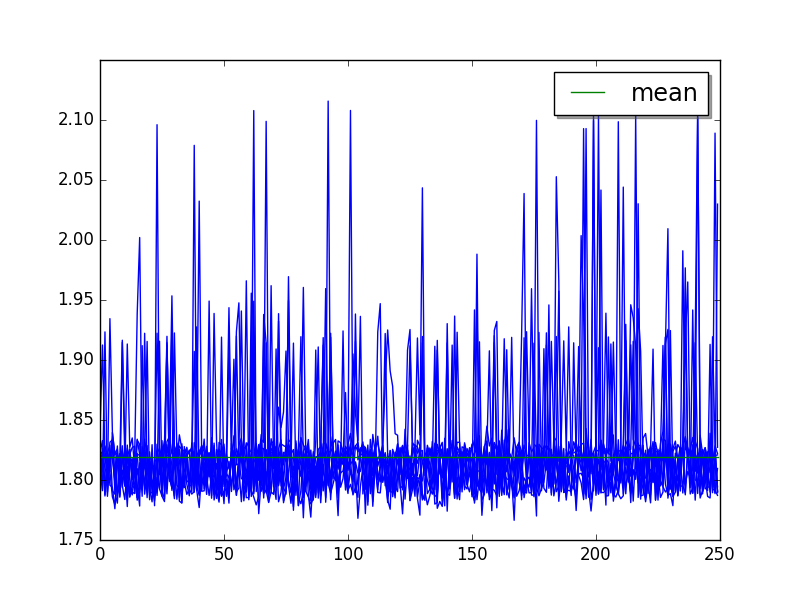Moving average (50 values) of runs: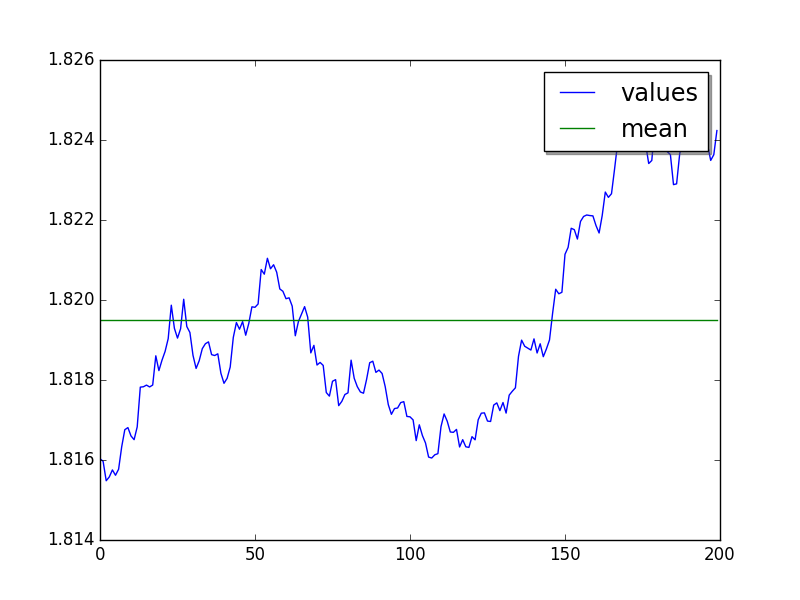## chameleon: loops=32, warmups=6, values=10¶

Overall: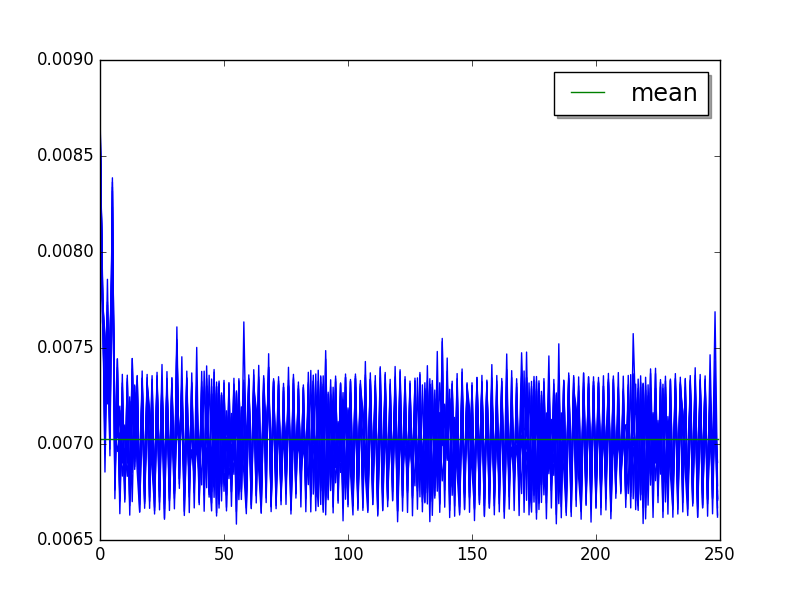Short cycle of 2 values (skip first 6 values, limit to 30 values):Large cycle of 42 values (Moving average 1 value, skip first 6 values):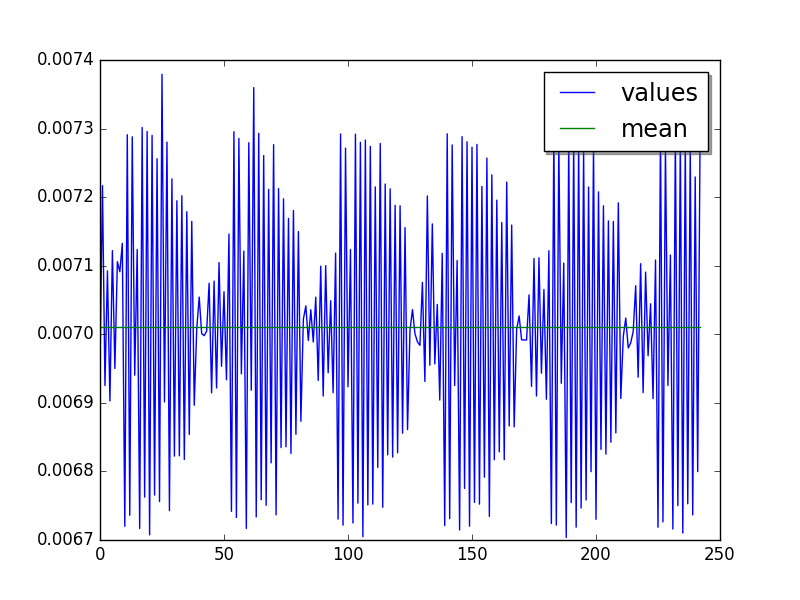Mean:

• loops=32, warmups=6, values=10: 7.05 ms +- 0.23 ms

• loops=32, warmups=6, values=42: 7.02 ms +- 0.20 ms

• LIMIT: loops=32, warmups=6: 7.01 ms +- 0.20 ms

## chaos: loops=16, warmups=20, values=25¶

Overall: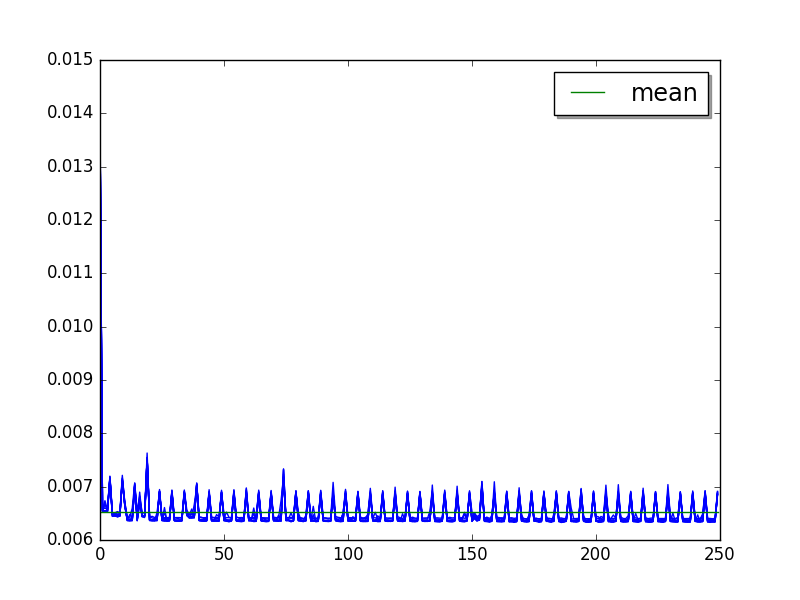Cycle of 5 values (average of runs, skip 20, limit to 50):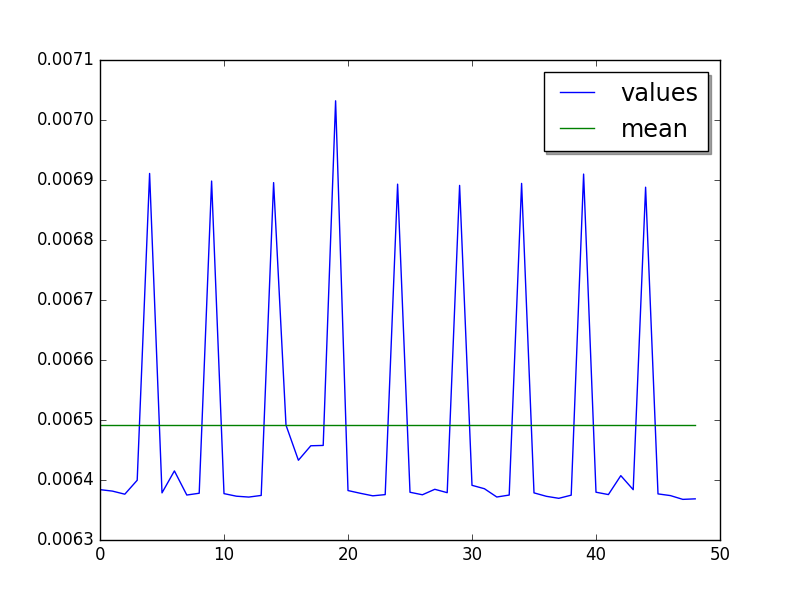Glitch at values 35..38.

Mean:

• loops=16, warmups=20, values=25: 6.50 ms +- 0.22 ms

• LIMIT: warmups=20: 6.48 ms +- 0.22 ms

## crypto_pyaes: loops=16, warmups=46, values=45¶

Overall: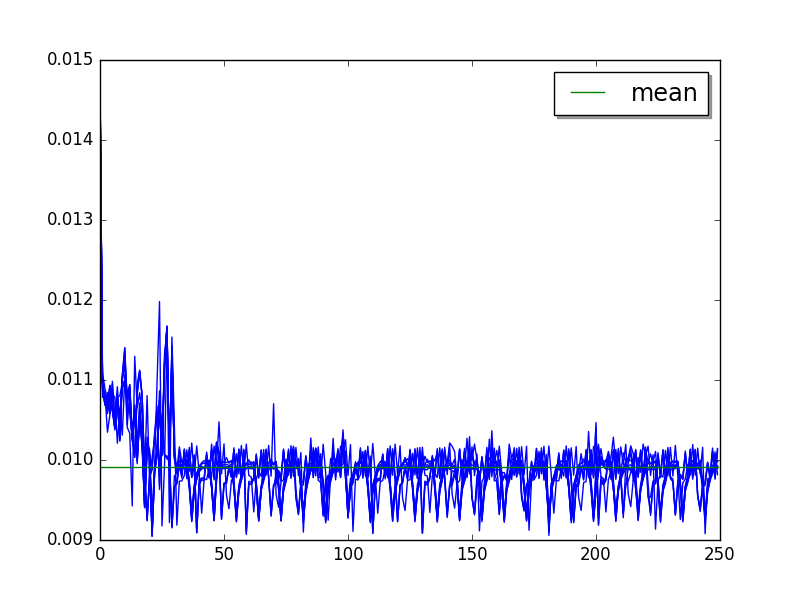Cycle of 9 values (average of runs, skip 46, limit to 45):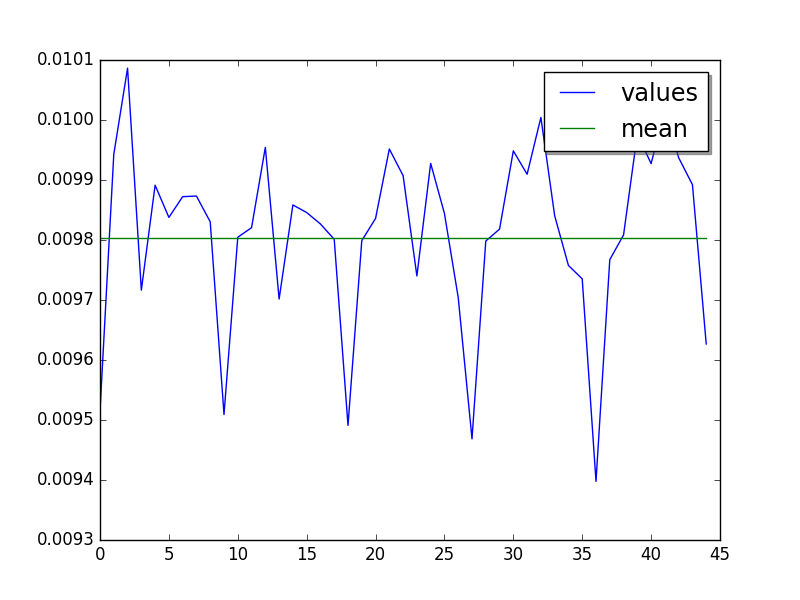Mean:

• loops=16, warmups=46, values=45: 9.81 ms +- 0.24 ms

• LIMIT: loops=16, warmups=46: 9.81 ms +- 0.24 ms

## deltablue: loops=256, warmups=14, values=55¶

Overall: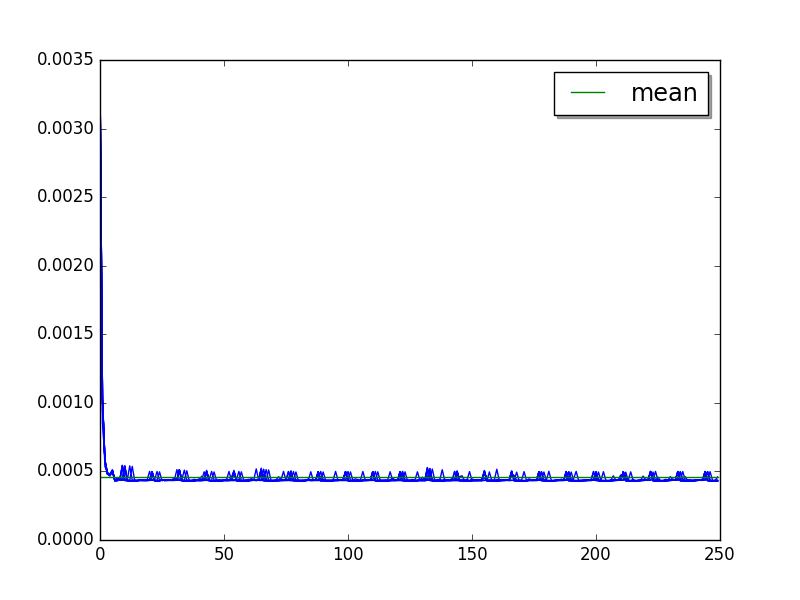Cycle of 11 values (average of runs, skip 14, limit to 55):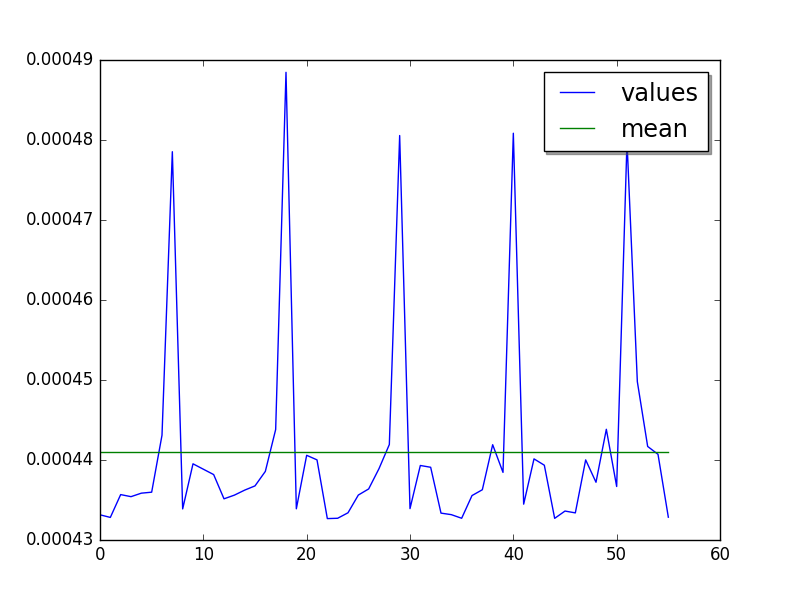Mean:

• loops=256, warmups=14, values=55: 441 us +- 20 us

• LIMIT: loops=256, warmups=14: 440 us +- 19 us

## django_template: loops=4, warmups=12, values=36¶

Overall: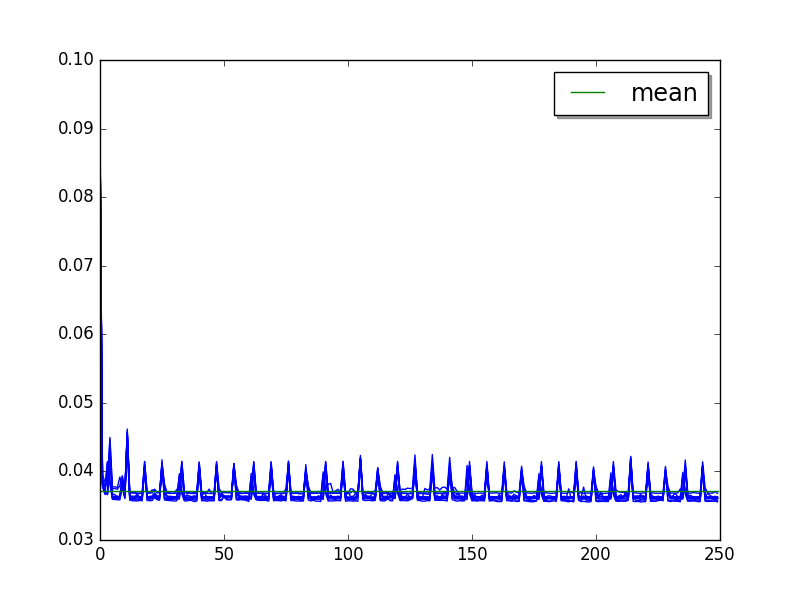Cycle of 7.3 values (average of runs, skip 12, limit to 36):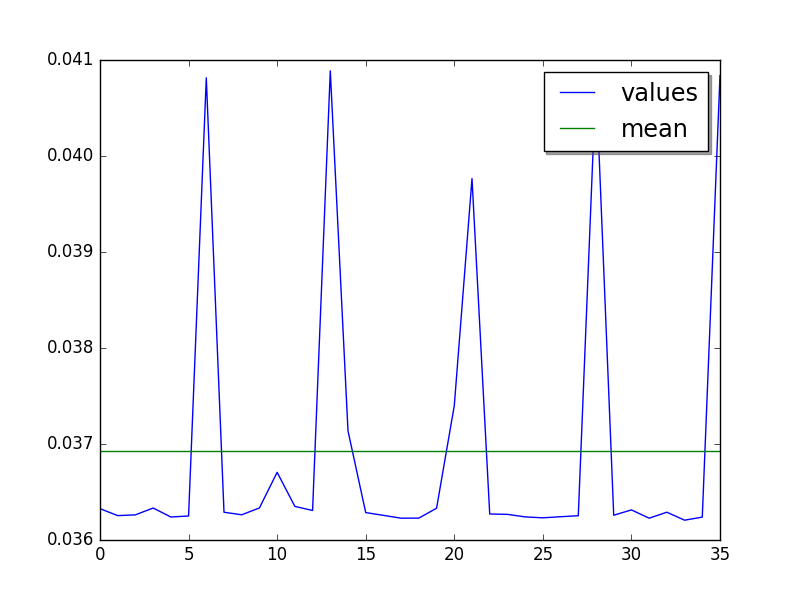Mean:

• loops=4, warmups=12, values=36: 36.9 ms +- 1.6 ms

• loops=4, warmups=12, values=73: 36.9 ms +- 1.6 ms

• LIMIT: loops=4, warmups=12: 36.9 ms +- 1.6 ms

## dulwich_log: loops=2, warmups=21, values=18¶

Overall: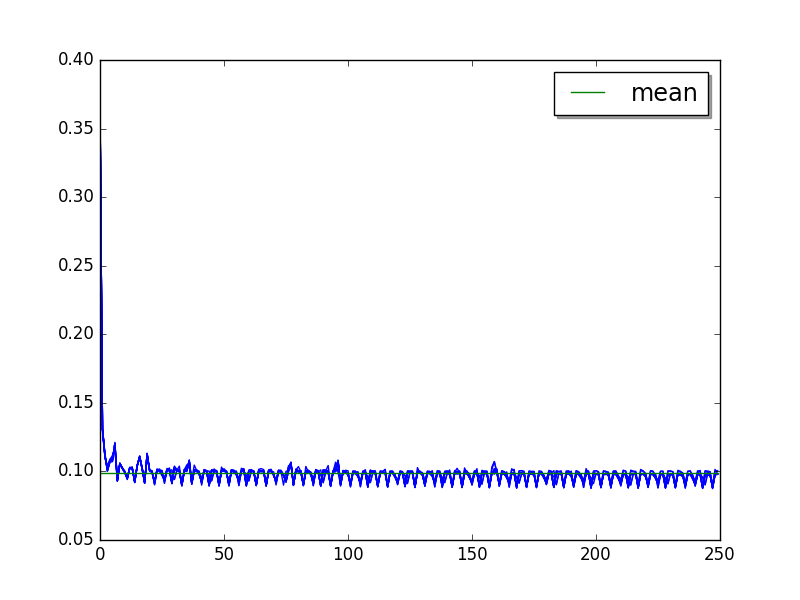Cycle of 3.6 values (average of runs, skip 21, limit to 18):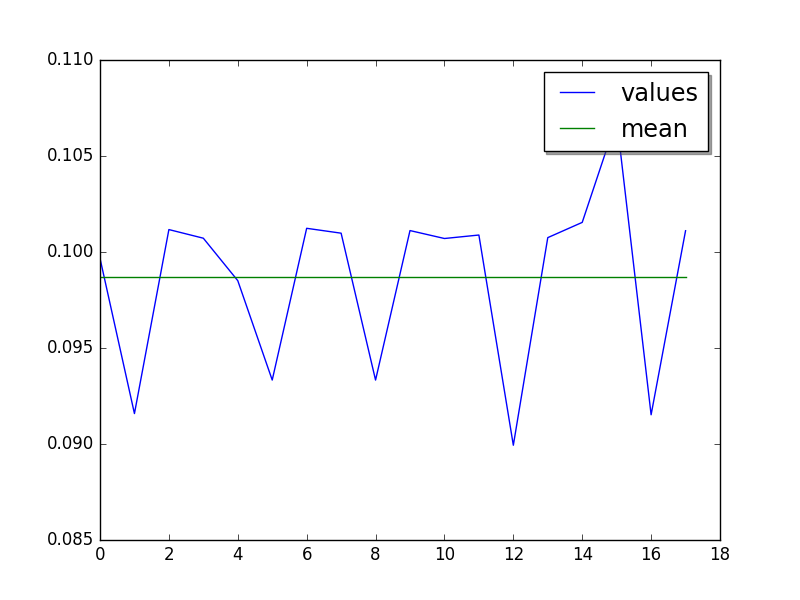Mean:

• loops=2, warmups=21, values=18: 98.6 ms +- 4.7 ms

• LIMIT: loops=2, warmups=21: 96.8 ms +- 4.4 ms

## fannkuch: loops=1, warmups=59, values=40¶

Overall: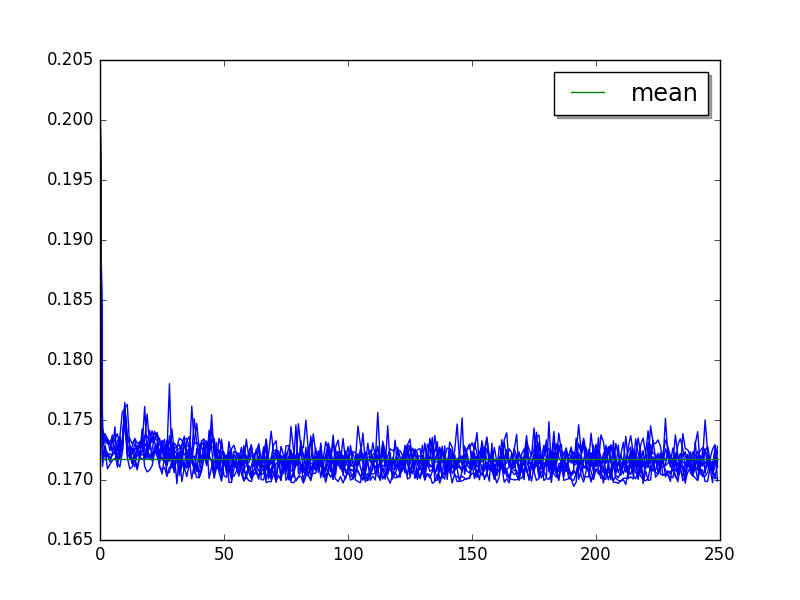Moving average of 25 values (skip 59), very small absolute variation (see the Y scale):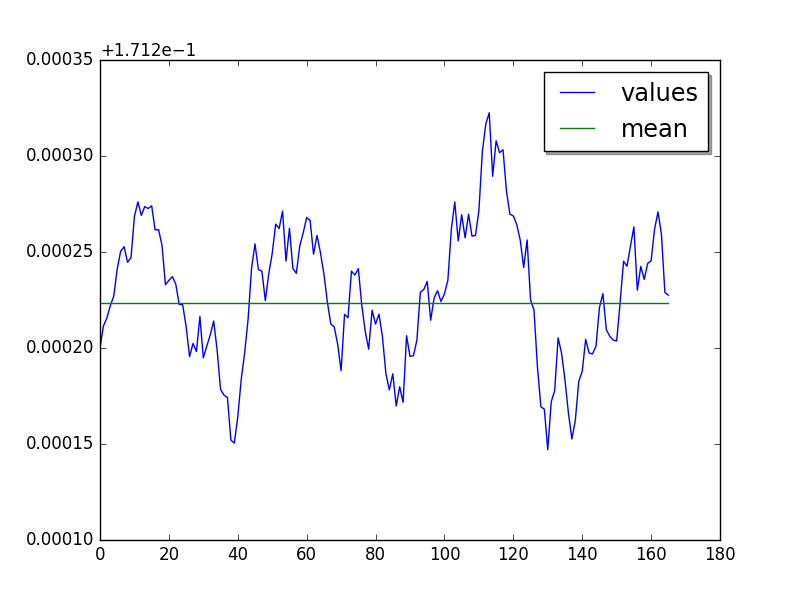Long cycle of 40 values. Not easy to see using moving average, spikes depend on the width of the moving window.

Mean:

• loops=1, warmups=59, values=40: 171 ms +- 1 ms

• LIMIT: loops=1, warmups=59: 171 ms +- 1 ms

## float: loops=4, warmups=25, values=40¶

Use the suboptimal step 2 to reduce total benchmark runtime, even if the step 3 is a little bit faster.

Overall: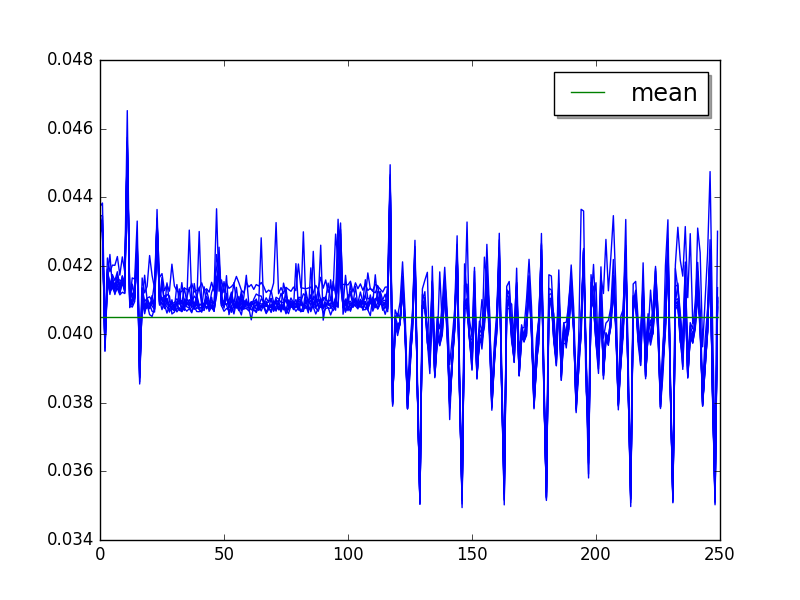Step 2, after warmup (average of runs, skip 25, limit 40):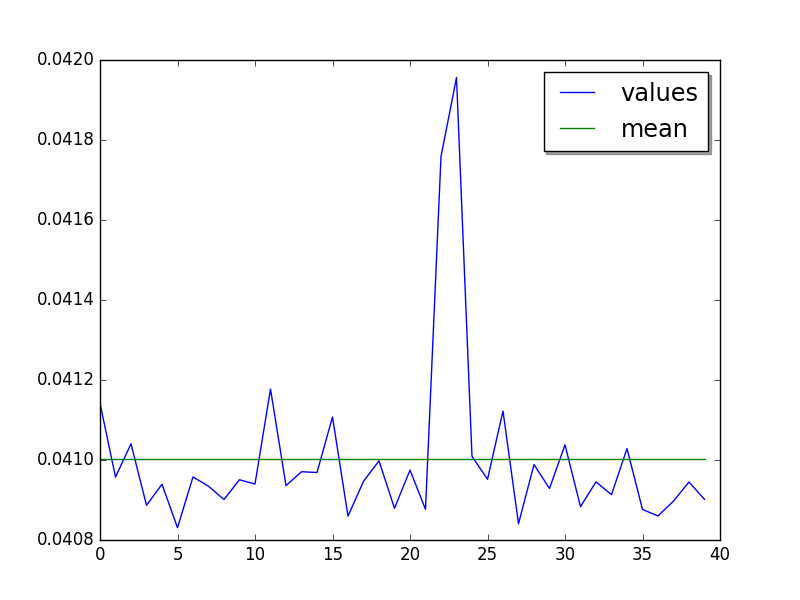Step 3: cycle of 16 values (average of runs, skip 119, limit 80):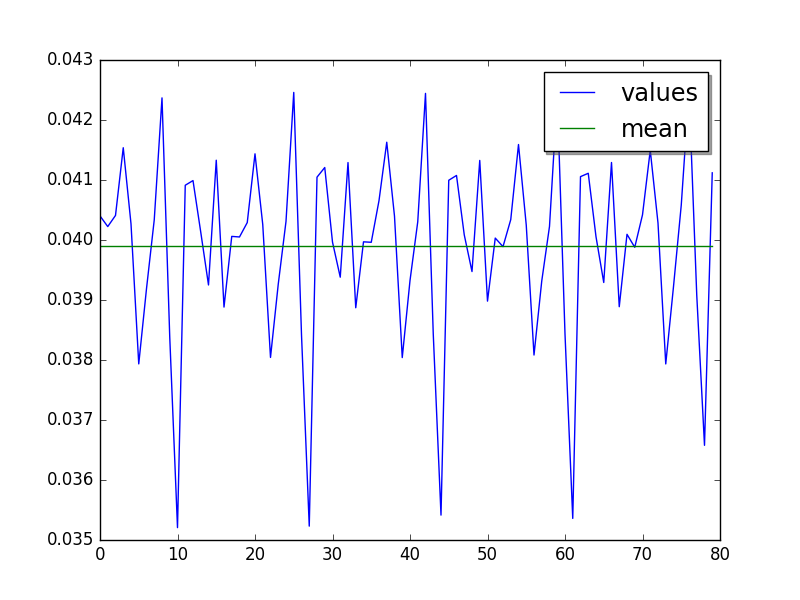Mean:

• Step 2: loops=4, warmups=25, values=40: 41.0 ms +- 0.4 ms

• Step 3: loops=4, warmups=119: 39.9 ms +- 1.6 ms

## genshi_text: loops=8, BUG!¶

BUG! 19 ms at value 0 => 92 ms at value 250, steady slowdown!

Overall: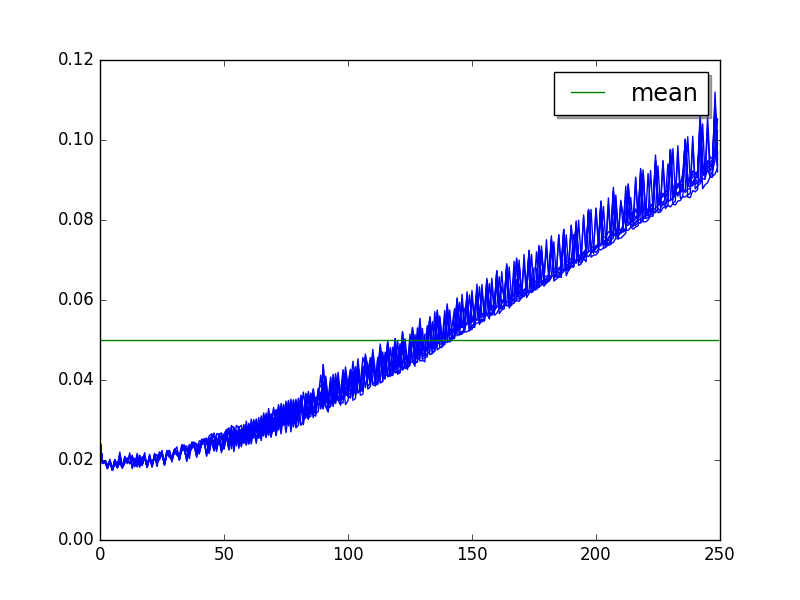## genshi_xml: loops=2, BUG!¶

BUG! 70 ms at value 0 => 200 ms at value 250, steady slowdown!

Overall: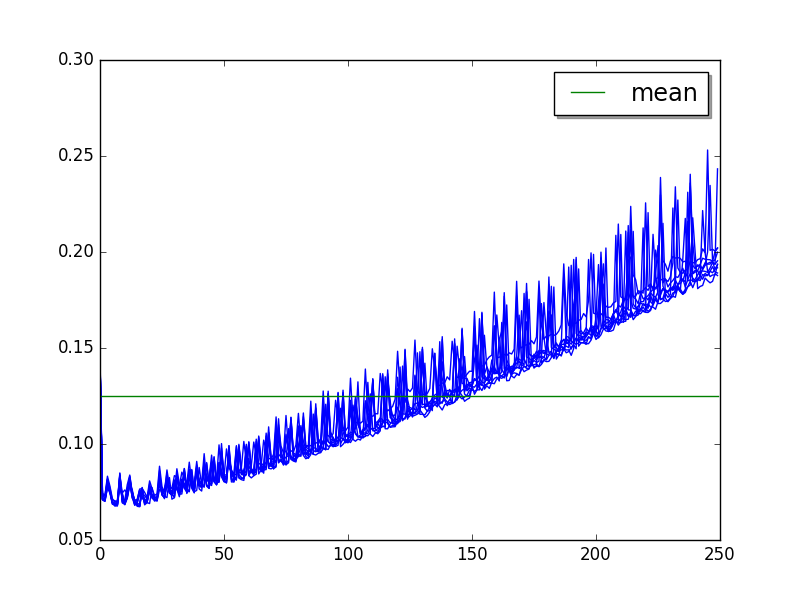## go: loops=2, warmups=87, values=80¶

Overall: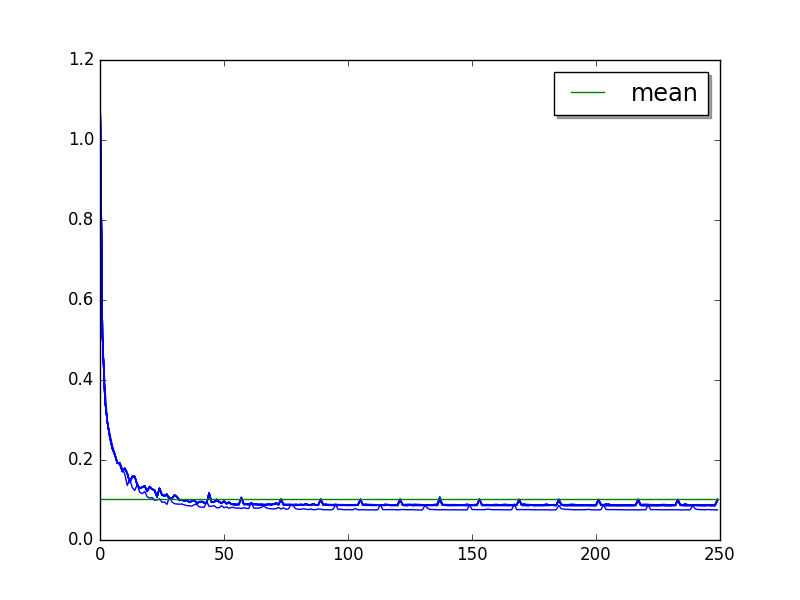Cycle of 5 values (average of runs, skip 87, limit 80):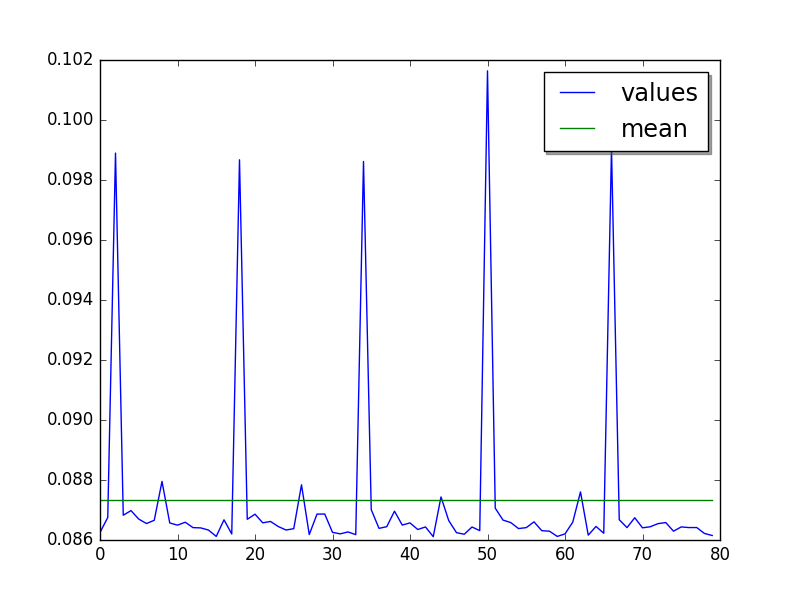Step 2 after warmup (skip 87):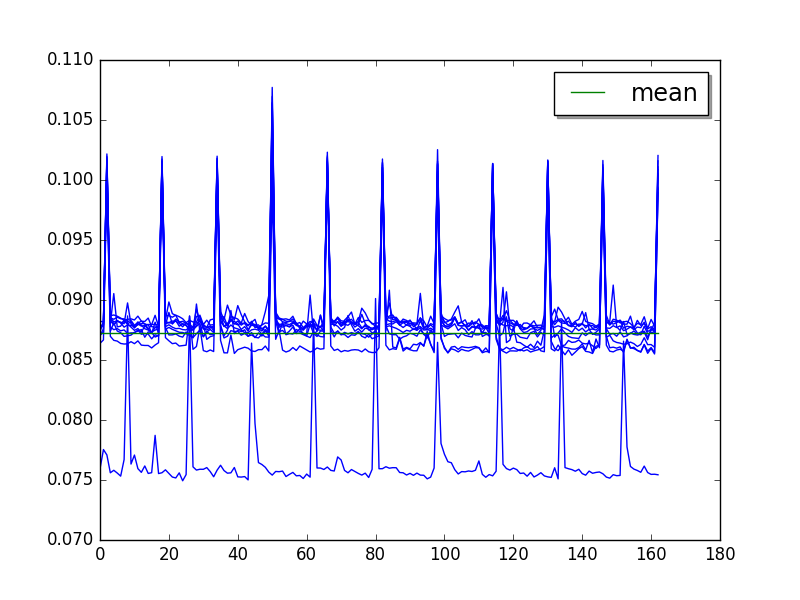Contiguous optimization (moving average of 50 values, skip 87), but only minor optimization (look at the Y scale):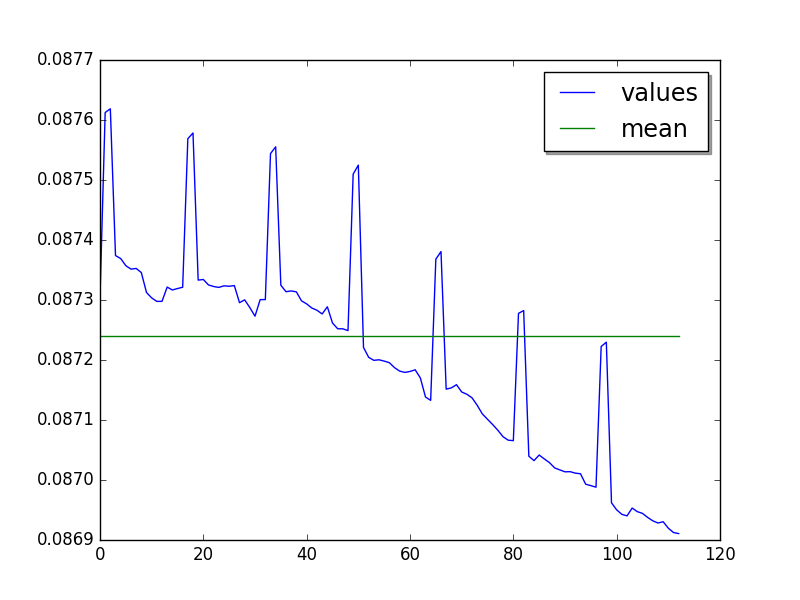Mean:

• loops=2, warmups=87, values=32: 87.4 ms +- 4.9 ms

• loops=2, warmups=87, values=80: 87.3 ms +- 5.0 ms

• LIMIT: loops=2, warmups=87: 87.2 ms +- 5.0 ms

## hexiom: loops=64, warmups=36, values=50¶

Only compute 2 cycles instead of 5 to limit the benchmark total runtime, since the cycle of long (25 values).

Overall: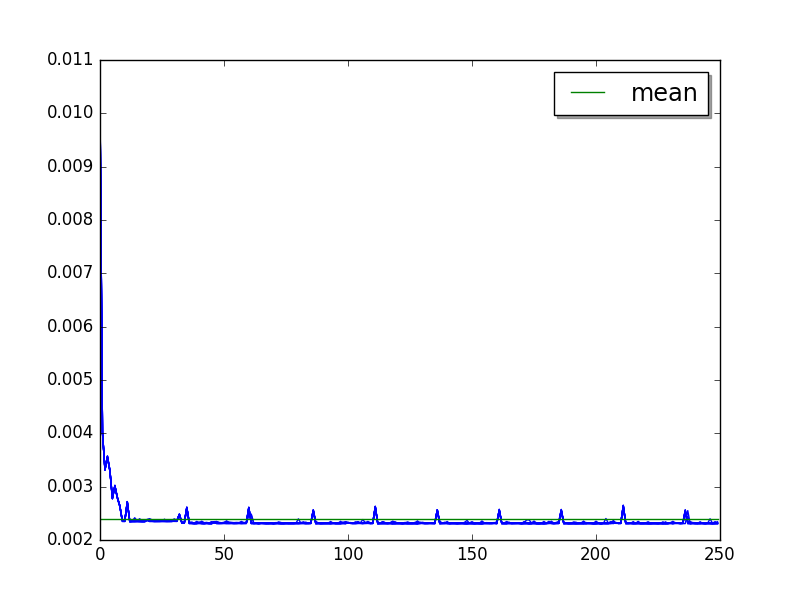Cycle of 25 values (average of runs, skip 36 , limit 127):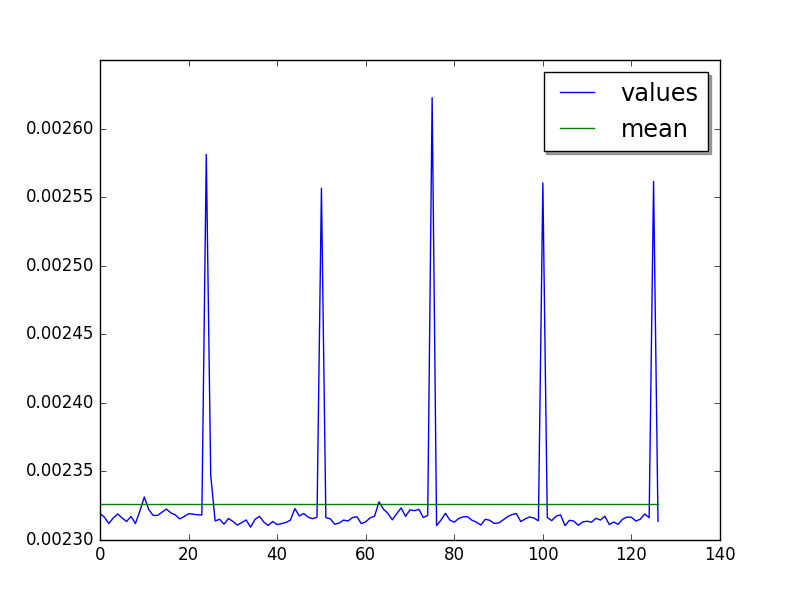Mean:

• loops=64, warmups=36, values=50: 2.32 ms +- 0.04 ms

• LIMIT: loops=64, warmups=36: 2.33 ms +- 0.05 ms

## hg_startup: loops=1, warmups=4, values=10¶

Overall: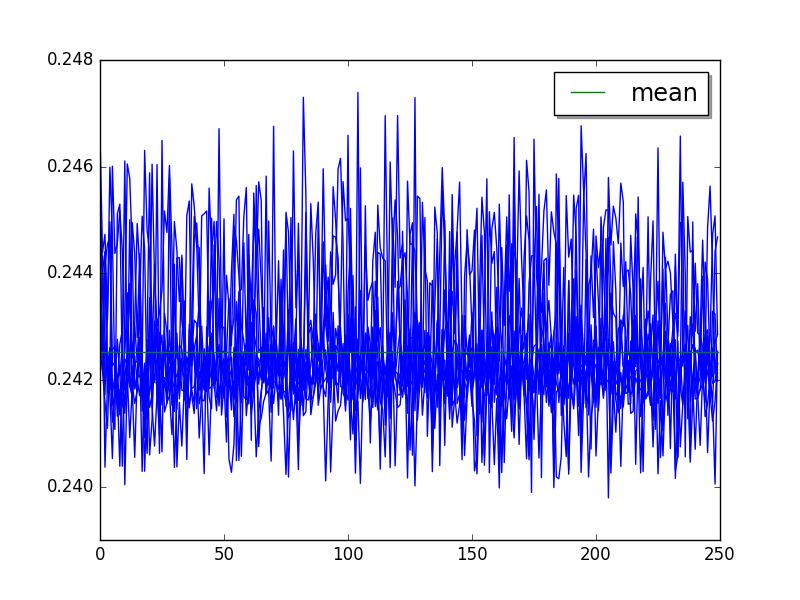Step 2 (skip 4, limit 10):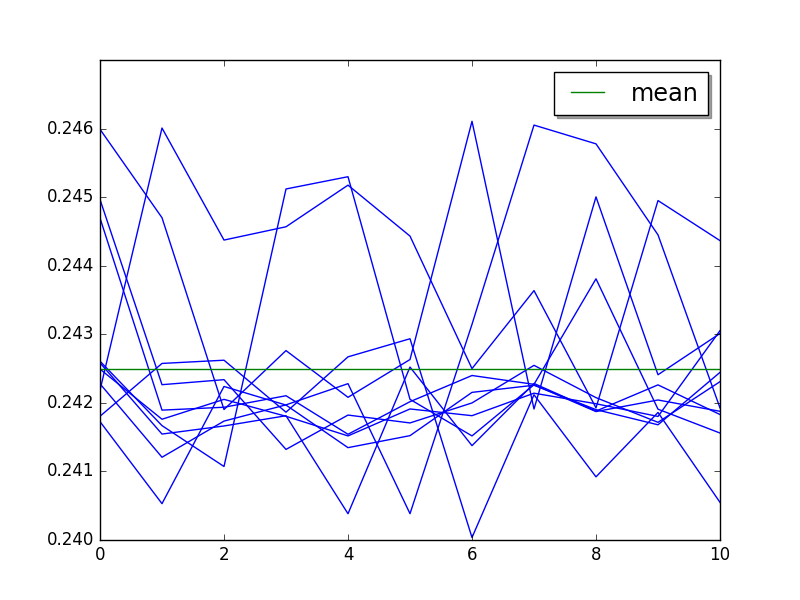Mean:

• loops=1, warmups=4, values=10: 243 ms +- 1 ms

• LIMIT: loops=1, warmups=4: 243 ms +- 1 ms

## html5lib: loops=2, warmups=50, values=50¶

On 250 values, it seems like PyPy optimizes the code multiple times. Tere are at least 3 steps:

• Warmup: 0..56

• Step 1: 57..158

• Step 2: 159..249

Use the step 1 which is suboptimal to reduce the benchmark total runtime.

Overall: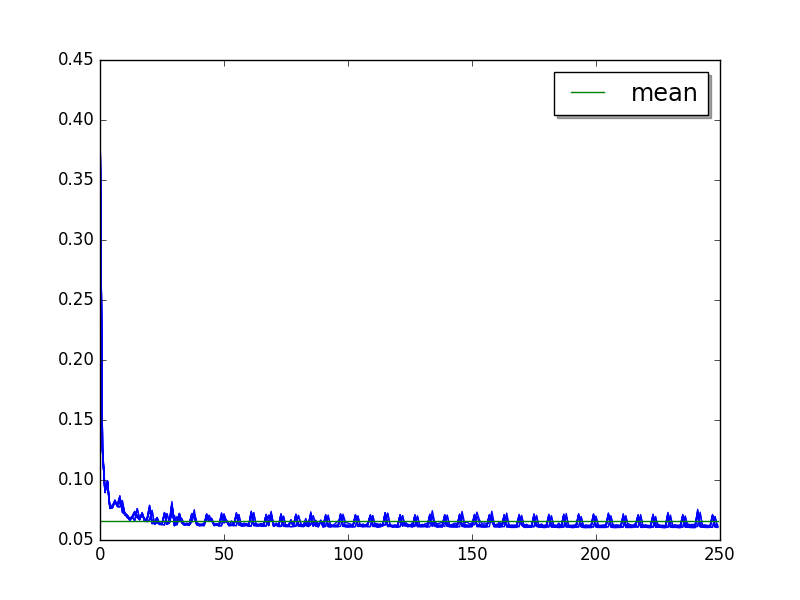Moving average of 25 values:Cycle (runs average, skip 57, limit 50):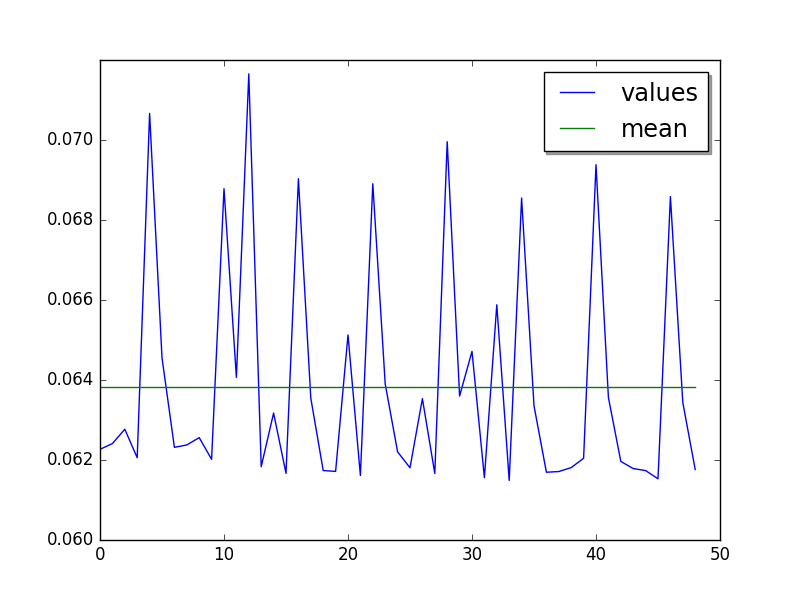Mean:

• loops=2, warmups=50, values=50: 63.8 ms +- 3.6 ms

• LIMIT: loops=2, warmups=50: 63.3 ms +- 3.5 ms

## json_dumps: loops=16, warmups=38, values=25¶

Overall: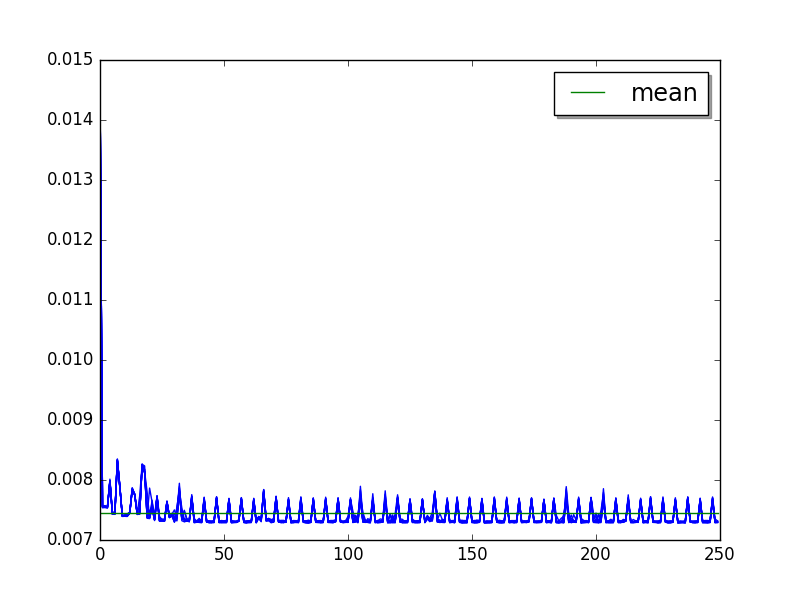Cycle of 5 values (runs average, skip 38, limit 50):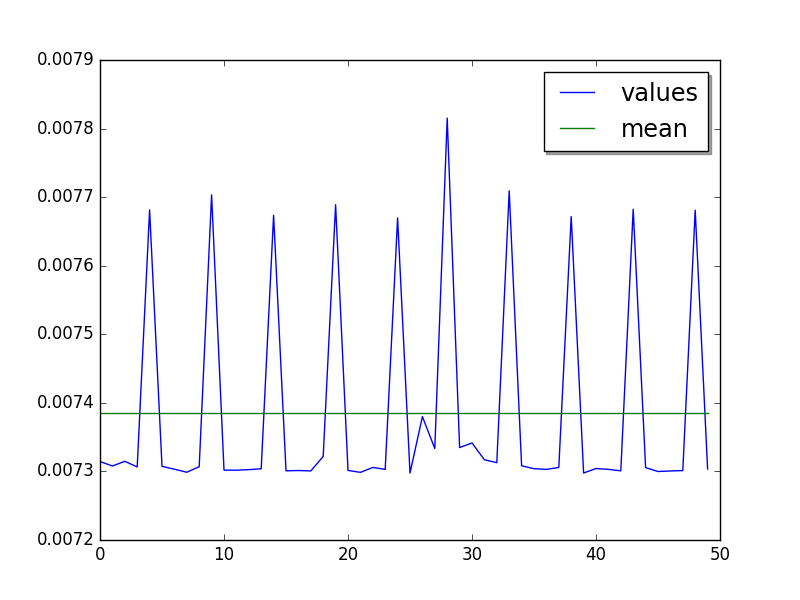Mean:

• Sample (5 cycles): loops=16, warmups=38, values=25: 7.38 ms +- 0.15 ms

• Sample (10 cycles): loops=16, warmups=38, values=50: 7.39 ms +- 0.16 ms

• LIMIT: loops=16, warmups=38: 7.39 ms +- 0.16 ms

Overall, +16% spike at value 90, +10% spike at value 190: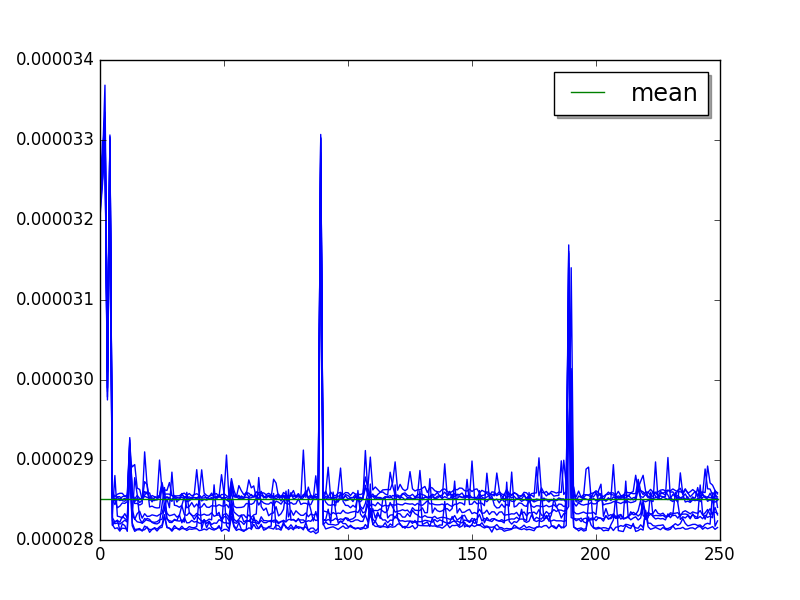Step 2 (skip 27, limit 50) with a pike at value 51: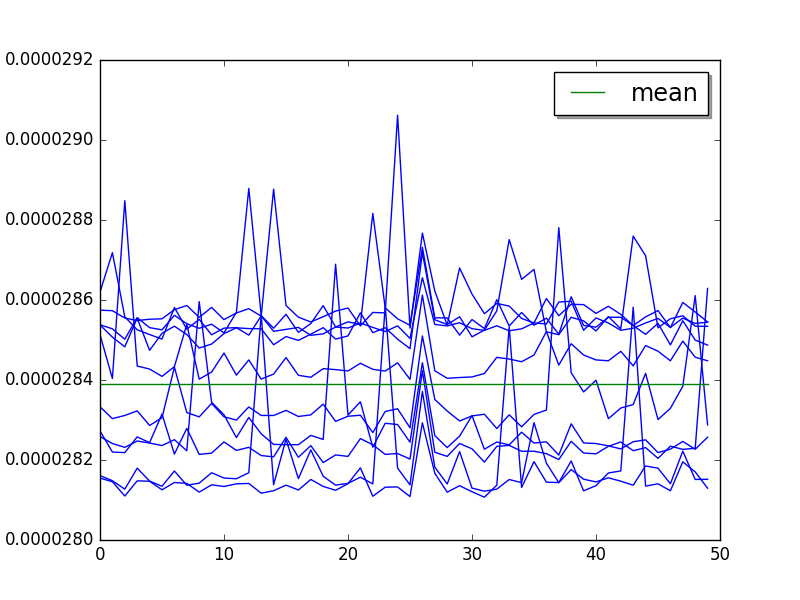Mean:

• Sample: loops=256, warmups=27, values=50: 28.4 us +- 0.2 us

• Limit: loops=256, warmups=27: 28.4 us +- 0.4 us

## TODO¶

• logging_format: loops=2048

• logging_silent: loops=134217728

• logging_simple: loops=4096

• mako: loops=8

• meteor_contest: loops=2

• nbody: loops=4

• nqueens: loops=4

• pathlib: loops=8

• pickle: loops=64

• pickle_dict: loops=64

• pickle_list: loops=256

• pickle_pure_python: loops=128

• pidigits: loops=1

• pyflate: loops=1

• python_startup: loops=8

• python_startup_no_site: loops=8

• raytrace: loops=8

• regex_compile: loops=2

• regex_dna: loops=1

• regex_effbot: loops=4

• regex_v8: loops=1

• richards: loops=64

• scimark_fft: loops=16

• scimark_lu: loops=64

• scimark_monte_carlo: loops=32

• scimark_sor: loops=128

• scimark_sparse_mat_mult: loops=1024

• spambayes: loops=4

• spectral_norm: loops=16

• sqlalchemy_declarative: loops=1

• sqlalchemy_imperative: loops=8

• sqlite_synth: loops=32768

• sympy_expand: loops=1

• sympy_integrate: loops=4

• sympy_sum: loops=2

• sympy_str: loops=1

• telco: loops=8

• unpack_sequence: loops=2048

• unpickle: loops=128

• unpickle_list: loops=1024

• unpickle_pure_python: loops=128

• xml_etree_parse: loops=1

• xml_etree_iterparse: loops=1

• xml_etree_generate: loops=2

• xml_etree_process: loops=2